Want to share your content on R-bloggers? click here if you have a blog, or here if you don't.

Check them out.

Here are thirty homoskedastic ones:

`> homo.wiener <- array(0, c(100, 30))> for (j in 1:30) {  for (i in 2:length(homo.wiener)) {          homo.wiener[i,j] <-  homo.wiener[ i - 1, j] + rnorm(1)                     }}`

`> for (j in 1:30) {`

`       plot( homo.wiener[,j],           type = "l", col = rgb(.1,.1,.1,.6),          ylab="", xlab="", ylim=c(-25,25)            );             par(new=TRUE) } `Here’s just the meat of that wiener, in case the `for` loops or window dressing were confusing.

`homo.wiener[i] <-  homo.wiener[ i - 1] + rnorm(1)`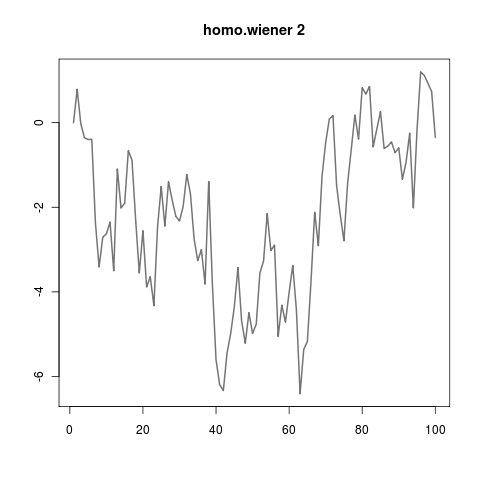I also made you some heteroskedastic wieners.

`> same for-loop encasing. ∀ j make wieners; ∀j plot wieners> hetero.wiener[i] <- hetero.wiener[ i-1 ] + rnorm(1, sd=rpois(1,1) )`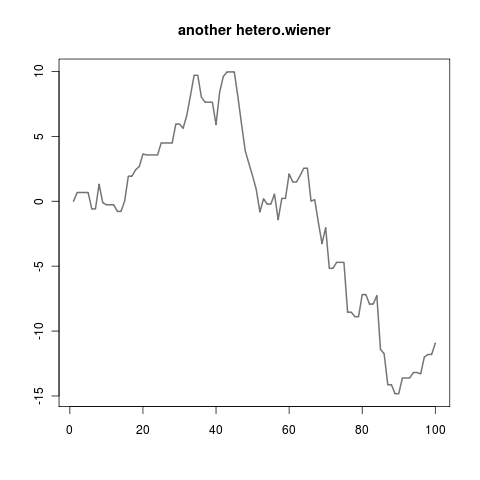It wasn’t even that hard — here are some autoregressive(1) wieners as well.

`> same for-loop encasing. ∀j make wieners; ∀j plot wieners> ar.wiener[i] <- ar.wiener[i-1]*.9 + rnorm(1)`Other types of wieners:

• `a.wiener[i-1] + rnorm(1) * a.wiener[i-1] + rnorm(1)`
• `central.limit.wiener[i-1] + sum( runif(17, min=-1) )`
• `cauchy.wiener[i-1] + rcauchy(1)      #leaping lizards!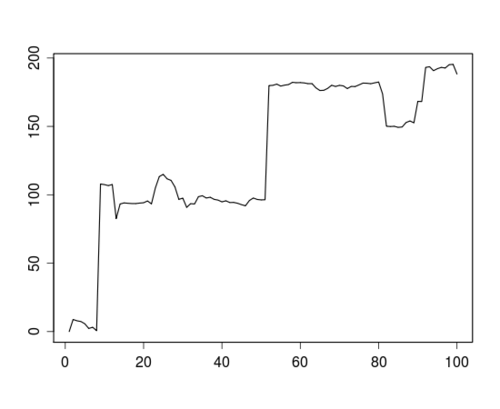`
• `random.eruption.wiener[i-1] + rnorm(1) * random.eruption.wiener[i-1] + rnorm(1)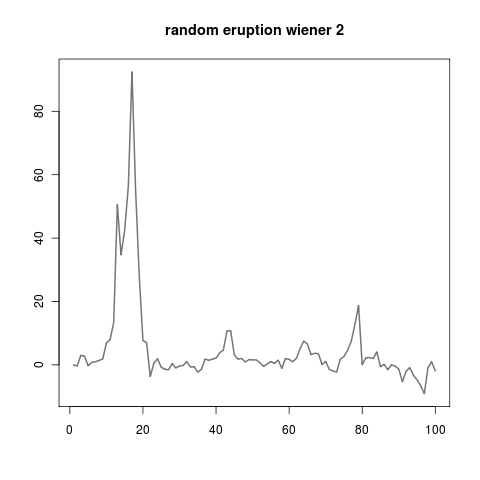`
• `non.markov.wiener[i-1] + non.markov.wiener[i-2] + rnorm(1)`
• `the.wiener.that.never.forgets[i] <- cumsum( the.wiener.that.never.forgets) + rnorm(1)`
• `non.wiener[i] <- rnorm(1)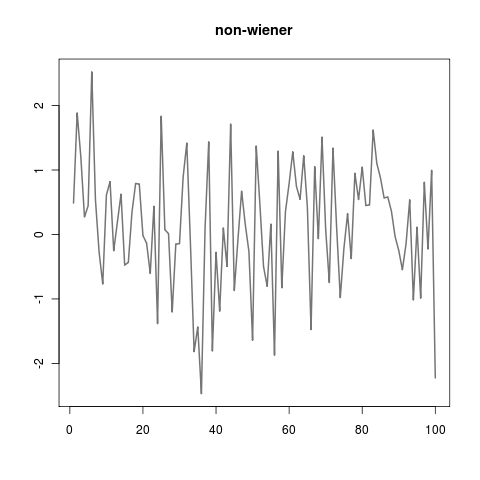`
• `moving.average.3.wiener[i] <- .6 * rnorm(n=1,sd=1) + .1 * rnorm(n=1,sd=50) + .3 * rnorm(n=1, mean=-3,sd=17)`
• `2d.wiener <- array(0, c(2, 100));ifelse( runif(1) > .5,     2d.wiener[1,i] <- 2d.wiener[1,i-1] + rnorm(1)             && 2d.wiener[2,i] <- 2d.wiener[2,i-1],     2d.wiener[2,i] <- 2d.wiener[2,i-1] + rnorm(1)             && 2d.wiener[ 1,i] <- 2d.wiener[1,i-1]`
• `131d.wiener <- array(0, c( 131, 100 )); ....`
• `cross.pollinated.wiener`
• contrasting `sd=1,2,3` of `homo.wieners`What really stands out in writing about these wieners after playing around with them, is that logically interesting wieners don’t always make for visually interesting wieners.

There are lots of games you can play with these wieners. Some of my favourites are:

• trying to make the wieners look like stock prices (I thought `sqrt(rcauchy(1))` errors with a little autocorrelation looked pretty good)
• trying to make them look like heart monitors

Also it’s pretty hard to tell which wieners are interesting just from looking at the codes above. I guess you will just have to go mess around with some wieners yourself. Some of them will surprise you and not do anything; that’s instructive as well.

VOICE OF GOD: WHAT’S UP. I AM THAT I AM. I DECLARE THAT THE WORD ‘WIENER’ IS OBJECTIVELY FUNNY. THAT’S ALL FOR NOW. SEE YOU WEDNESDAY THE 17TH.